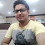### How to create common event handler function to handle and respond to multiple buttons and perform the operations like sum, subtraction, multiplication and divisionIntroduction: In this article i am going to share the trick to calculate the operations like Sum, subtraction, multiplication and division on two numbers by creating a common event handler method in asp.net using both C# and VB languages.
Note: The real benefit of this is that we don't need to write the code on each button, we can create a single common function that handle and respond to all the buttons to perform their specific task.

Description: While working on asp.net project i was required to perform different calculation but without writing the code on each button so i searched on internet and after some efforts got the solution hint to create a common event handler function that can do the job.  So i created the common handler and going to share that will all so that developers can take the advantage of this.

Implementation: Let's create a simple web application to perform the operations like Sum, subtraction, multiplication and division on two numbers.

Asp.Net C# Section:

• Design the asp.net page (default.aspx) as:
<fieldset style="width: 400px;">
<legend>Use of common handler in Asp.net C#</legend>
<table>
<tr>
<td>Enter First Number: </td>
<td><asp:TextBox ID="txtFirstNum" runat="server"></asp:TextBox>
</td>
</tr>
<tr>
<td>Enter Second Number: </td>
<td><asp:TextBox ID="txtSecondNum" runat="server"></asp:TextBox></td>
</tr>
<tr>
<td>Result: </td>
<td><asp:TextBox ID="txtResult" runat="server"></asp:TextBox></td>
</tr>
<tr>
<td>&nbsp;</td>
<td>
<asp:Button ID="btnSum" runat="server" onclick="Calculate" Text="Sum" />
<asp:Button ID="btnSub" runat="server" onclick="Calculate" Text="Subtract" />
<asp:Button ID="btnMul" runat="server" onclick="Calculate" Text="Multiply"/>
<asp:Button ID="btnDiv" runat="server" onclick="Calculate" Text="Divide"/>
</td>
</tr>
</table>
</fieldset>

Note: Have you noticed the highlighted part in above Html markup. All the button are calling the same event handler on click of button.

• Now its time to create the common event handler function so in the Code behind file (default.aspx.cs)  create a common function that will respond to all the buttons:
protected void Calculate(object sender, EventArgs e)
{
if (sender == btnSum)
{
txtResult.Text = Convert.ToString(Convert.ToInt32(txtFirstNum.Text) + Convert.ToInt32(txtSecondNum.Text));
}
else if (sender == btnSub)
{
txtResult.Text = Convert.ToString(Convert.ToInt32(txtFirstNum.Text) - Convert.ToInt32(txtSecondNum.Text));
}
else if (sender == btnMul)
{
txtResult.Text = Convert.ToString(Convert.ToInt32(txtFirstNum.Text) * Convert.ToInt32(txtSecondNum.Text));
}
else
{
txtResult.Text = Convert.ToString(Convert.ToInt32(txtFirstNum.Text) / Convert.ToInt32(txtSecondNum.Text));
}
}

Asp.Net VB Section

• Design the asp.net page (default.aspx) as:
<fieldset style="width:400px;">
<legend>Use of common handler in Asp.net C#</legend>
<table>
<tr>
<td>Enter First Number: </td>
<td><asp:TextBox ID="txtFirstNum" runat="server"></asp:TextBox></td>
</tr>
<tr>
<td>Enter Second Number: </td>
<td><asp:TextBox ID="txtSecondNum" runat="server"></asp:TextBox></td>
</tr>
<tr>
<td>Result: </td>
<td><asp:TextBox ID="txtResult" runat="server"></asp:TextBox></td>
</tr>
<tr>
<td>&nbsp;</td>
<td>
<asp:Button ID="btnSum" runat="server" Text="Sum" />
<asp:Button ID="btnSub" runat="server" Text="Subtract" />
<asp:Button ID="btnMul" runat="server" Text="Multiply"/>
<asp:Button ID="btnDiv" runat="server" Text="Divide"/>
</td>
</tr>ot
</table>
</fieldset>

• In the Code behind file (default.aspx.cs)  write the code as:
Protected Sub btnSum_Click(ByVal sender As Object, ByVal e As System.EventArgs) Handles btnSum.Click, btnSub.Click, btnMul.Click, btnDiv.Click
If sender Is btnSum Then
txtResult.Text = Convert.ToInt32(txtFirstNum.Text) + Convert.ToInt32(txtSecondNum.Text)
ElseIf sender Is btnSub Then
txtResult.Text = Convert.ToInt32(txtFirstNum.Text) - Convert.ToInt32(txtSecondNum.Text)
ElseIf sender Is btnMul Then
txtResult.Text = Convert.ToInt32(txtFirstNum.Text) * Convert.ToInt32(txtSecondNum.Text)
Else
txtResult.Text = Convert.ToInt32(txtFirstNum.Text) / Convert.ToInt32(txtSecondNum.Text)
End If
End Sub

Note: The same button is handling the events of all the button

Now over to you:
" I hope you have got the trick to create common event handler function to handle and respond to multiple buttons in asp.net and If you like my work; you can appreciate by leaving your comments, hitting Facebook like button, following on Google+, Twitter, Linked in and Pinterest, stumbling my posts on stumble upon and subscribing for receiving free updates directly to your inbox . Stay tuned and stay connected for more technical updates."
Previous
Next Post »

Unknown